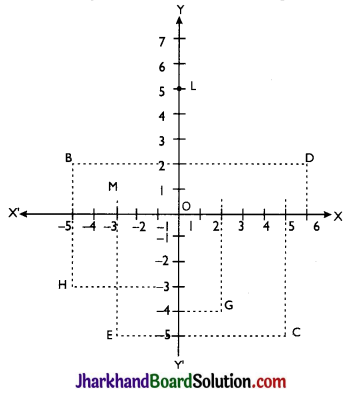# JAC Class 9 Maths Solutions Chapter 3 Coordinate Geometry Ex 3.2

Jharkhand Board JAC Class 9 Maths Solutions Chapter 3 Coordinate Geometry Ex 3.2 Textbook Exercise Questions and Answers.

## JAC Board Class 9th Maths Solutions Chapter 3 Coordinate Geometry Ex 3.2

Page – 61

Question 1.
Write the answer of each of the following questions:
(i) What is the name of horizontal and the vertical lines drawn to determine the position of any point in the Cartesian plane?
(ii) What is the name of each part of the plane formed by these two lines?
(iii) Write the name of the point where these two lines intersect.
(i) The names of horizontal line and vertical line drawn to determine the position of any point in the Cartesian plane are x-axis and y-axis respectively.
(ii) The name of each part of the plane formed by these two lines x-axis and y-axis is quadrant.
(iii) The point where these two lines intersect is called origin.Question 2.
See Fig., and write the following:(i) The coordinates of B.
(ii) The coordinates of C.
(iii) The point identified by the coordinates (-3, -5).
(iv) The point identified by the coordinates (2, -4).
(v) The abscissa of the point D.
(vi) The ordinate of the point H.
(vii) The coordinates of the point L.
(viii)The coordinates of the point M.